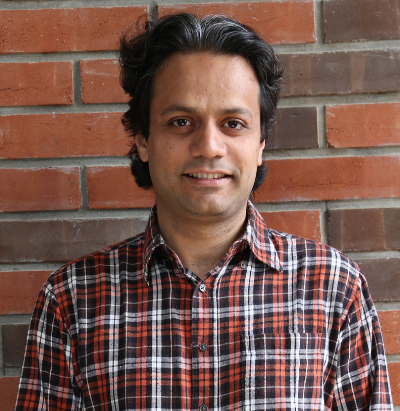## Quantum Field Theory 1 – Introduction and Foundations

This course will introduce a number of important concepts and foundational topics in the relativistic quantum theory of fields. The focus will be on the hamiltonian formulation of QFT, spin 0 (scalar) and spin 1/2 (spinor) fields, and on simple tree-level processes for the most part.

Course Outline

Quantum Mechanics + Special relativity, the need for many particles, antiparticles and quantum fields.

Fock Space, many particle QM, the quantum field as coupled harmonic oscillators.

Classical Field Theory: Lagrangians, Hamiltonians and equation of motion, Noether’s theorem, Symmetries (spacetime and internal), conserved currents, energy-momentum tensor, Canonical Quantisation.

Relativistic causality, cluster decomposition and locality, mode expansions.

Perturbation theory and Scattering: Schrodinger, Heisenberg and Dirac pictures, Dyson formula and Lorentz invariant S - matrix, Wick contractions and normal ordering, simple tree level processes in the canonical operator formalism. Green’s functions, Propagators, LSZ and KL representation, Feynman rules.

QFT in curved spacetime - a primer.

Spinorial representations of the Lorentz Group. Weyl Spinors and Weyl equation. Dirac equation and plane wave solutions, quantisation of the Dirac field, anti-commutation relations, Majorana fermions. Propagator and Feynman diagrams and Feynman rules.

Basic Quantum Electrodynamics (Feynman rules and amplitudes for simple tree level processes).

#### Physics